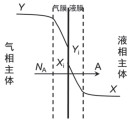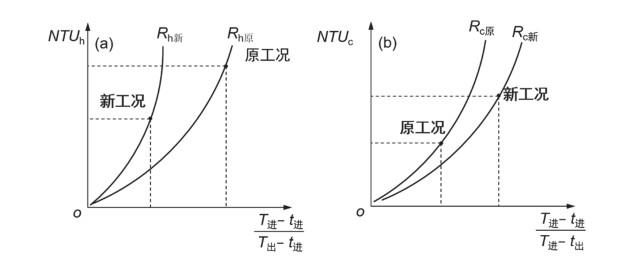## Enlightenment of Mass Transfer on Heat Transfer Teaching in Teaching of Principles of Chemical Engineering and Its Application

Du Zhiping, Yin Xia, Ding Yigang, Liu Shengpeng, Xiao Yanhua,

 基金资助: 武汉工程大学校级重点项目.  X2018001Abstract

The common points of absorption and heat transfer were found by comparison of the assumptions and transfer model. Furthermore, the computational formula and graphs of the number and the length of a transfer unit were deduced in the countercurrent heat transfer through learning from the treatment method of absorption. Based on the study, the basic analysis methodology for qualitative analysis of operational heat transfer problems was proposed. The connection between mass transfer and heat transfer was established through the comparison, so that they were no longer random knowledge points, which was beneficial for students to understand and master principles of chemical engineering.

Keywords： Heat transfer ; Mass transfer ; Number of transfer unite ; Qualitative analysis

Du Zhiping. Enlightenment of Mass Transfer on Heat Transfer Teaching in Teaching of Principles of Chemical Engineering and Its Application. University Chemistry[J], 2020, 35(12): 261-267 doi:10.3866/PKU.DXHX201910047

### 1.2 吸收与传热的传递模型比较

 吸收 传热双模理论模型图 传热模型图 ①气-液接触的相间有稳定的相界面；②相界面两侧各有一层虚拟膜(有效膜)，膜内为层流，传质以分子扩散方式进行；③传质阻力全部集中在虚拟膜内，膜外流体高度湍流，传质阻力为零；④相界面上气液处于平衡状态。 ①金属壁面两侧各有一层虚拟膜(有效膜)，膜内为层流，膜内的传热以热传导方式进行；②传热阻力全部集中在虚拟膜内，膜外流体高度湍流，传热阻力为零；③金属壁内以热传导方式进行。

${N_{\text{A}}} = {k_Y}(Y - {Y_{\text{i}}}) = {k_X}({X_{\text{i}}} - X) = {K_Y}(Y - {Y_{\text{e}}})$

$q = {\alpha _{\text{h}}}(T - {T_{\text{W}}}) = {\alpha _{\text{c}}}({t_{\text{W}}} - t) = K(T - t)$

### 2.1 传热的操作线和图解

${q_{m, {\text{h}}}}{c_{p, {\text{h}}}}{\text{d}}T = {q_{m, {\text{c}}}}{c_{p, {\text{c}}}}{\text{d}}t$

### 图1$T=\frac{{q}_{m, \text{c}}{c}_{p, \text{c}}}{{q}_{m, \text{h}}{c}_{p, \text{h}}}t+({T}_{出}-\frac{{q}_{m, \text{c}}{c}_{p, \text{c}}}{{q}_{m, \text{h}}{c}_{p, \text{h}}}t{}_{进})$

### 图2①操作线线上任一点的坐标P(t, T)代表了换热器内该截面上冷、热流体的温度；

②操作线上任一点P与平衡线间的垂直距离或水平距离(Tt)为换热器内该截面上的传热推动力；

③两线间垂直距离(Tt)或水平距离(Tt)的变化显示了传热过程推动力沿换热面的变化规律。

### 2.2 传热的基本方程

${q_{\text{h}}}{\text{d}}{A_{\text{h}}} = {\alpha _{\text{h}}}(T - {T_{\text{W}}}){\text{d}}{A_{\text{h}}} = {q_{\text{c}}}{\text{d}}{A_{\text{c}}} = {\alpha _{\text{c}}}({t_{\text{W}}} - t){\text{d}}{A_{\text{c}}} = \frac{\lambda }{b}({T_{\text{W}}} - {t_{\text{W}}}) = K(T - t){\text{d}}A$

$\frac{1}{{{K_{\text{h}}}}} = \frac{1}{{{\alpha _{\text{h}}}}} + \frac{b}{\lambda } \times \frac{{{d_{\text{h}}}}}{{{d_{\text{m}}}}} + \frac{1}{{{\alpha _{\text{c}}}}} \times \frac{{{d_{\text{h}}}}}{{{d_{\text{c}}}}}或\frac{1}{{{K_{\text{c}}}}} = \frac{1}{{{\alpha _{\text{h}}}}} + \frac{b}{\lambda } \times \frac{{{d_{\text{c}}}}}{{{d_{\text{m}}}}} + \frac{1}{{{\alpha _{\text{c}}}}} \times \frac{{{d_{\text{c}}}}}{{{d_{\text{h}}}}}$

$A=\frac{{q}_{m, \text{h}}{c}_{p, \text{h}}}{K}{\int }_{{T}_{出}}^{{T}_{进}}\frac{{\text{d}}T}{T-t}}=\frac{{q}_{m, \text{c}}{c}_{p, \text{c}}}{K}{\int }_{{t}_{进}}^{{t}_{出}}\frac{{\text{d}}t}{T-t}$

$L=\frac{{q}_{m, \text{h}}{c}_{p, \text{h}}}{K\text{π}d}{\int }_{{T}_{出}}^{{T}_{进}}\frac{{\text{d}}T}{T-t}}=\frac{{q}_{m, \text{c}}{c}_{p, \text{c}}}{K\text{π}d}{\int }_{{t}_{进}}^{{t}_{出}}\frac{{\text{d}}t}{T-t}$

$T-t=(1-\frac{{q}_{m, \text{h}}{c}_{p, \text{h}}}{{q}_{m, \text{c}}{c}_{p, \text{c}}})T+\frac{{q}_{m, \text{h}}{c}_{p, \text{h}}}{{q}_{m, \text{c}}{c}_{p, \text{c}}}{T}_{出}-{t}_{进}$

$\begin{array}{l}NT{U}_{\text{h}}=\frac{1}{1-{R}_{\text{h}}}\mathrm{ln}[(1-{R}_{\text{h}})\frac{{T}_{进}-{t}_{进}}{{T}_{出}-{t}_{进}}+{R}_{\text{h}}]{}}_{}^{}({R}_{\text{h}}\ne 1)\\ NT{U}_{\text{h}}=\frac{{T}_{进}-{T}_{出}}{{T}_{出}-{t}_{进}}{}}_{}^{}({R}_{\text{h}}=1)\end{array$

$\begin{array}{l}NT{U}_{\text{c}}=\frac{1}{1-{R}_{\text{c}}}\mathrm{ln}[(1-{R}_{\text{c}})\frac{{T}_{进}-{t}_{进}}{{T}_{进}-{t}_{出}}+{R}_{\text{c}}]{}}_{}^{}\ \ \ ({R}_{\text{c}}\ne 1)\\ NT{U}_{\text{c}}=\frac{{t}_{出}-{t}_{进}}{{T}_{出}-{t}_{进}}{}}_{}^{}({R}_{\text{c}}=1)\end{array$

### 图31)由图3(a)可知，当RhTt一定，横坐标(Tt)/( Tt)的值越大，热流体的出口温度越低，完成任务所需的NTUh越多，热流体的传热效率高，因此横坐标(Tt)/( Tt)反映了传热效率的大小，其值越大，传热效率越高。

2) 图3(a)中，Rh可变形为Rh = 1/[(qm, c·cp, c)/(qm, h·cp, h)]，而传热平衡线的斜率为1，所以Rh反映了平衡线斜率与操作线斜率的比值。当(Tt)/( Tt)、Tt一定，Rh值越小，操作线的斜率大，根据图2两线相距越远，传热推动力越大，越有利于传热过程，所需的传热单元数越少，故Rh越小，曲线越平坦。同时Rh值的大小也影响流体出口温度的极限值：

①当Rh < 1，即操作线斜率大于平衡线斜率时，若换热器的管长为无限长，则冷流体入口端优先达到到平衡(见图4(a))，热流体的最低出口温度接近于冷流体的入口温度，Tt，因此，如果需要尽可能地降低热流体的温度，宜采用Rh < 1的操作。

### 图4(a) Rh < 1；(b) Rh > 1

②当Rh > 1，即操作线的斜率小于平衡线斜率时，若换热器的管长为无限长，则热流体入口端优先达到平衡(见图4(b))，冷流体的最高出口温度接近于热流体的入口温度，tT，因此，如果需要尽可能地提高冷流体的温度，宜采用Rh > 1的操作。

③当Rh = 1，即操作线的斜率等于平衡线的斜率时，若换热器的管长为无限长，则冷、热流体入口端同时达到平衡，TttT

3) 图3(b)图3(a)类似，(Tt)/(Tt)反映了冷流体的传热效率，其值越大，冷流体出口温度越高，传热效率越高；Rc反映了操作线斜率与平衡线斜率的比值，其值越大，曲线越陡。

## 3 传热过程分析的思路

①根据条件确定LTUhRh的变化趋势；

②用L = LTUh·NTUh确定NTUh的变化趋势；

③用传质单元数法确定T的变化趋势；

t的分析：一是利用热量守恒分析；二是根据条件确定LTUcRc的变化趋势，进而由L = LTUc·NTUc确定NTUc的变化趋势，最后用传质单元数法确定t的变化趋势。

①传热过程中：

${K_{\text{h}}} \propto q_{m, {\text{h}}}^n(n < 0.8)$；又$LT{U_{\text{h}}} = \frac{{{q_{m, {\text{h}}}}{c_{p, {\text{h}}}}}}{{Kπd}} \propto q_{m, {\text{h}}}^{1 - n}$；当热流体的质量流量qm, h增加时，LTUh也随之增加。

②由L = LTUh·NTUh可知：L不变，LTUh增加，则NTUh降低。

③由于Rh = (qm, h·cp, h)/(qm, c·cp, c)也随qm, h的增加而增加；由图5(a)可知：相对于原工况，新工况左移，所以(Tt)/( Tt)减小；又tT不变，所以T增加。

### 图5(a)热流体；(b)冷流体

2)冷流体出口的温度变化趋势。

Kc = Kh(dc/dh)，则KcKh的增加而增加；又LTUc = (qm, ccp, c)/(Kcπd)，qm, c不变，所以LTUc降低。

②由L = LTUc·NTUc可知：L不变，LTUc降低，则NTUc增加。

③由于Rc = (qm, c·cp, c)/(qm, h·cp, h)也随qm, h的增加而降低；由图5(b)可知：相对于原工况，新工况左移，所以(Tt)/( Tt)增加；又tT不变，所以t增加。

## 4 结语

1)由于吸收和传热假设的相似，使它们具有相似的操作线方程，这样传热也能建立与吸收类似的操作线和平衡线的图解关系。从图解关系中能直观了解传热面任意一处的推动力大小，以及其沿换热面的变化规律。

2)吸收和传热都采用了将传递阻力全部集中于有效膜内，膜内为层流，总阻力为各部分阻力和的建模方式，从而奠定了传质与传热可相互贯通的基础。借鉴传质单元数法的方法和特点，将换热面积的计算分解为传热单元长度和传热单元数，推导出了它们的计算公式，绘制出了传热单元数的基本图形，并以此为基础建立了分析传热操作型问题的基本方法。

3)通过比较传质与传热找出了它们在建模方法、公式求取和图解，以及应用分析上的共同点，实现了传质与传热的相互贯通，这样不同的传递过程之间不再是看上去独立的、分散的知识点，而是前后彼此可以相互串联、相互启发、相互印证的，有助于学生对化工原理知识的理解、掌握和升华。

## 参考文献 原文顺序 文献年度倒序 文中引用次数倒序 被引期刊影响因子

McCabe, W. L.; Smith, J. C.; Harriott P. Unit Operations of Chemical Engineering.伍钦,钟理,夏清,熊丹柳,改编.第7版.北京:化学工业出版社, 2015.

/

 〈〉